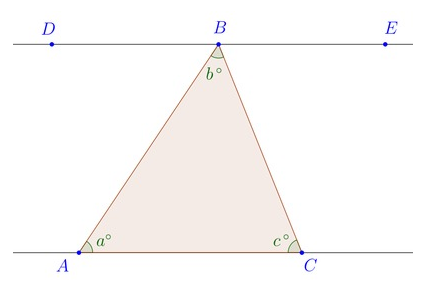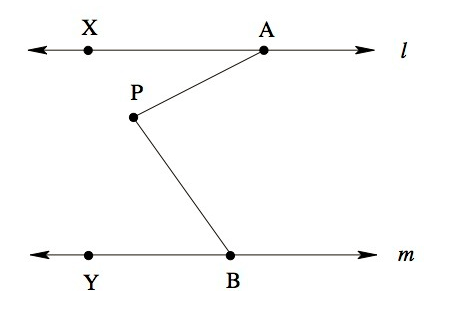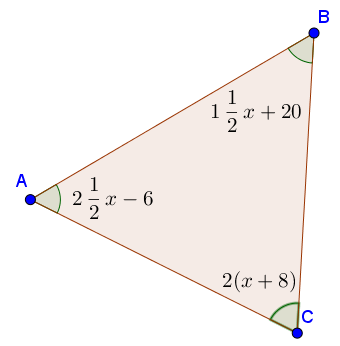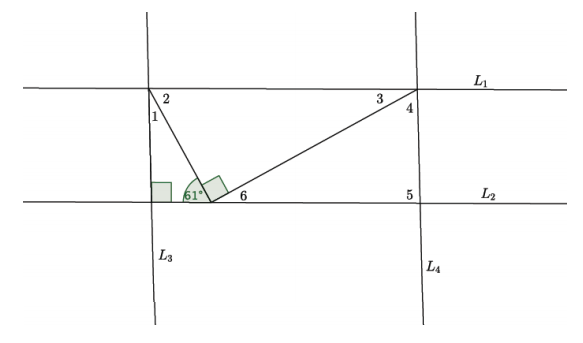Match Fishtank is now Fishtank Learning!

# Transformations and Angle Relationships

## Objective

Define and use the interior angle sum theorem for triangles.

## Common Core Standards

### Core Standards

?

• 8.G.A.5 — Use informal arguments to establish facts about the angle sum and exterior angle of triangles, about the angles created when parallel lines are cut by a transversal, and the angle-angle criterion for similarity of triangles. For example, arrange three copies of the same triangle so that the sum of the three angles appears to form a line, and give an argument in terms of transversals why this is so.

?

• 7.G.A.2

• 7.G.B.5

## Criteria for Success

?

1. Know that the sum of the interior angles of a triangle is always 180°.
2. Prove that the sum of the interior angles of a triangle equals 180° by lining the three angles on a straight line.
3. Use the interior angle sum theorem and parallel line properties of angles to determine additional angle relationships.

## Tips for Teachers

?

Students have experience working with triangles and angle measurements from seventh grade when they investigated unique triangles. While students may know that the angles in a triangle add up to 180°, in this lesson they have the chance to prove this fact using parallel line angle relationships.

#### Remote Learning Guidance

If you need to adapt or shorten this lesson for remote learning, we suggest prioritizing Anchor Problem 1 (benefits from discussion) and Anchor Problem 2 (benefits from worked example). Find more guidance on adapting our math curriculum for remote learning here.

#### Fishtank Plus

• Problem Set
• Student Handout Editor
• Vocabulary Package

## Anchor Problems

?

### Problem 1

Given that ${\overleftrightarrow{DE} \parallel \overleftrightarrow{AC}}$ in the diagram below, prove that ${a+b+c=180}$.#### References

Illustrative Mathematics A Triangle's Interior Angles

A Triangle's Interior Angles, accessed on Aug. 10, 2017, 9:48 a.m., is licensed by Illustrative Mathematics under either the CC BY 4.0 or CC BY-NC-SA 4.0. For further information, contact Illustrative Mathematics.

Modified by Fishtank Learning, Inc.

### Problem 2

In the picture below, lines $l$ and $m$ are parallel. The measure of $\angle PAX$ is ${31^{\circ}}$, and the measure of $\angle PBY$ is ${54^{\circ}}$.

What is the measure of $\angle APB$?#### References

Illustrative Mathematics Find the Missing Angle

Find the Missing Angle, accessed on Oct. 13, 2017, 4:22 p.m., is licensed by Illustrative Mathematics under either the CC BY 4.0 or CC BY-NC-SA 4.0. For further information, contact Illustrative Mathematics.

### Problem 3

Determine the angle measures of each interior angle in the triangle.## Problem Set

?The following resources include problems and activities aligned to the objective of the lesson that can be used to create your own problem set.

• Have students create their own triangle and prove that the sum of the interior angles is 180°.

?

In the diagram below, ${L_1 \parallel L_2}$ and ${L_3 \parallel L_4}$. Use the diagram to answer the questions that follow.1. What is the measure of angle 1? Show how you determine your answer.
2. What other answers are congruent to angle 1?
3. What is the measure of angle 4? Show how you determine your answer.

#### References

EngageNY Mathematics Grade 8 Mathematics > Module 2 > Topic C > Lesson 13Exit Ticket

Grade 8 Mathematics > Module 2 > Topic C > Lesson 13 of the New York State Common Core Mathematics Curriculum from EngageNY and Great Minds. © 2015 Great Minds. Licensed by EngageNY of the New York State Education Department under the CC BY-NC-SA 3.0 US license. Accessed Dec. 2, 2016, 5:15 p.m..

Modified by Fishtank Learning, Inc.

?